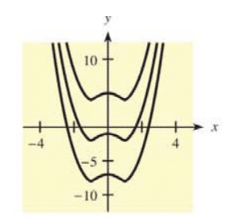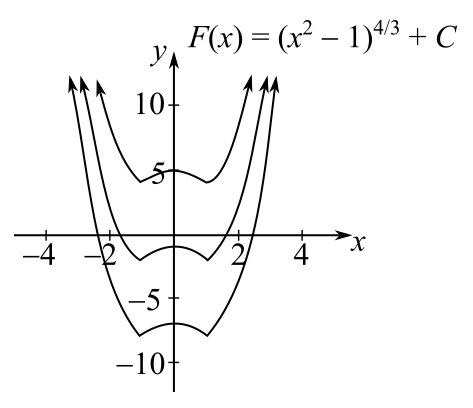Chapter 12.2, Problem 41E### Mathematical Applications for the ...

11th Edition
Ronald J. Harshbarger + 1 other
ISBN: 9781305108042

#### Solutions

Chapter
Section### Mathematical Applications for the ...

11th Edition
Ronald J. Harshbarger + 1 other
ISBN: 9781305108042
Textbook Problem

# In each of Problems 43 and 44, a family of functions is given, together with the graphs of some functions in the family. Write the indefinite integral that gives the family. F ( x ) = ( x 2 − 1 ) 4 / 3 + CTo determine

To calculate: The indefinite integral that gives the family of the curves if F(x)=(x21)4/3+C.Explanation

Given Information:

The provided equation is,

F(x)=(x21)4/3+C

And, the family of curves is,

Formula used:

According to the power formula of integrals, if u=u(x), then:

undu=un+1n+1+C

Calculation:

Consider the provided function,

F(x)=(x21)4/3+C

Now, to obtain the indefinite integral that gives the above family of curves, first differentiate both sides with respect to x as,

ddx(F(x))=ddx((x21)4/3

### Still sussing out bartleby?

Check out a sample textbook solution.

See a sample solution

#### The Solution to Your Study Problems

Bartleby provides explanations to thousands of textbook problems written by our experts, many with advanced degrees!

Get Started

#### f(x)1x(x+2)(x3)

Applied Calculus for the Managerial, Life, and Social Sciences: A Brief Approach

#### Find the matrix A such that A= Hint: Let A=[abcd]

Finite Mathematics for the Managerial, Life, and Social Sciences

#### True or False: The graph of x = 5 is a cylinder.

Study Guide for Stewart's Multivariable Calculus, 8th

#### An integral for the solid obtained by rotating the region at the right about the x-axis is:

Study Guide for Stewart's Single Variable Calculus: Early Transcendentals, 8th